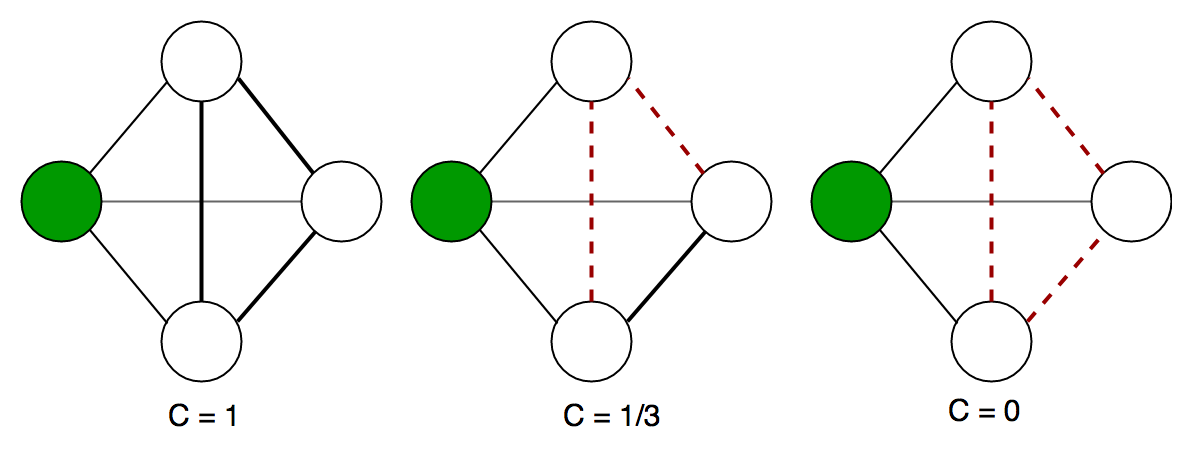Open in App
Not now

# Clustering Coefficient in Graph Theory

• Difficulty Level : Basic
• Last Updated : 31 Oct, 2022

In graph theory, a clustering coefficient is a measure of the degree to which nodes in a graph tend to cluster together. Evidence suggests that in most real-world networks, and in particular social networks, nodes tend to create tightly knit groups characterized by a relatively high density of ties; this likelihood tends to be greater than the average probability of a tie randomly established between two nodes (Holland and Leinhardt, 1971; Watts and Strogatz, 1998).

Two versions of this measure exist: the global and the local. The global version was designed to give an overall indication of the clustering in the network, whereas the local gives an indication of the embeddedness of single nodes.

Global clustering coefficient:

The global clustering coefficient is based on triplets of nodes. A triplet consists of three connected nodes. A triangle therefore includes three closed triplets, one centered on each of the nodes (n.b. this means the three triplets in a triangle come from overlapping selections of nodes). The global clustering coefficient is the number of closed triplets (or 3 x triangles) over the total number of triplets (both open and closed). The first attempt to measure it was made by Luce and Perry (1949). This measure gives an indication of the clustering in the whole network (global), and can be applied to both undirected and directed networks.

Local clustering coefficient:

A graphformally consists of a set of vertices V and a set of edges E between them. An edgeconnects vertexwith vertex. The neighborhoodfor a vertexis defined as its immediately connected neighbors as follows:We defineas the number of vertices,, in the neighborhood,, of a vertex. The local clustering coefficientfor a vertexis then given by the proportion of links between the vertices within its neighborhood divided by the number of links that could possibly exist between them. For a directed graph,is distinct from, and therefore for each neighborhoodthere arelinks that could exist among the vertices within the neighborhood (is the number of neighbors of a vertex). Thus, the local clustering coefficient for directed graphs is given asAn undirected graph has the property thatandare considered identical. Therefore, if a vertexhasneighbors,edges could exist among the vertices within the neighborhood. Thus, the local clustering coefficient for undirected graphs can be defined asLetbe the number of triangles onfor undirected graph G. That is,is the number of sub-graphs of G with 3 edges and 3 vertices, one of which is v. Letbe the number of triples on. That is,is the number of sub-graphs (not necessarily induced) with 2 edges and 3 vertices, one of which is v and such that v is incident to both edges. Then we can also define the clustering coefficient as lueIt is simple to show that the two preceding definitions are the same, since. These measures are 1 if every neighbor connected tois also connected to every other vertex within the neighborhood, and 0 if no vertex that is connected toconnects to any other vertex that is connected toExample local clustering coefficient on an undirected graph. The local clustering coefficient of the green node is computed as the proportion of connections among its neighbours. Here is the code to implement the above clustering coefficient in a graph. It is a part of the networkx library and can be directly accessed using it.

## Python

 def average_clustering(G, trials=1000):    """Estimates the average clustering coefficient of G.     The local clustering of each node in G is the    fraction of triangles that actually exist over    all possible triangles in its neighborhood.    The average clustering coefficient of a graph    G is the mean of local clustering.     This function finds an approximate average    clustering coefficient for G by repeating n    times (defined in trials) the following    experiment: choose a node at random, choose    two of its neighbors at random, and check if    they are connected. The approximate coefficient    is the fraction of triangles found over the    number of trials _.     Parameters    ----------    G : NetworkX graph     trials : integer        Number of trials to perform (default 1000).     Returns    -------    c : float        Approximated average clustering coefficient.           """    n = len(G)    triangles = 0    nodes = G.nodes()    for i in [int(random.random() * n) for i in range(trials)]:        nbrs = list(G[nodes[i]])        if len(nbrs) < 2:            continue        u, v = random.sample(nbrs, 2)        if u in G[v]:            triangles += 1    return triangles / float(trials)

Note: The above code is valid for undirected networks and not for the directed networks. The code below has been run on IDLE(Python IDE of windows). You would need to download the networkx library before you run this code. The part inside the curly braces represent the output. It is almost similar as Ipython(for Ubuntu users).

## Python

 >>> import networkx as nx>>> G=nx.erdos_renyi_graph(10,0.4)>>> cc=nx.average_clustering(G)>>> cc#Output of Global CC0.08333333333333333>>> c=nx.clustering(G)>>> c# Output of local CC{0: 0.0, 1: 0.3333333333333333, 2: 0.0, 3: 0.0, 4: 0.0, 5: 0.0, 6: 0.0,7: 0.3333333333333333, 8: 0.0, 9: 0.16666666666666666}

The above two values give us the global clustering coefficient of a network as well as local clustering coefficient of a network. Next into this series, we will talk about another centrality measure for any given network.

This article is contributed by Jayant Bisht. If you like GeeksforGeeks and would like to contribute, you can also write an article using write.geeksforgeeks.org or mail your article to review-team@geeksforgeeks.org. See your article appearing on the GeeksforGeeks main page and help other Geeks.

My Personal Notes arrow_drop_up